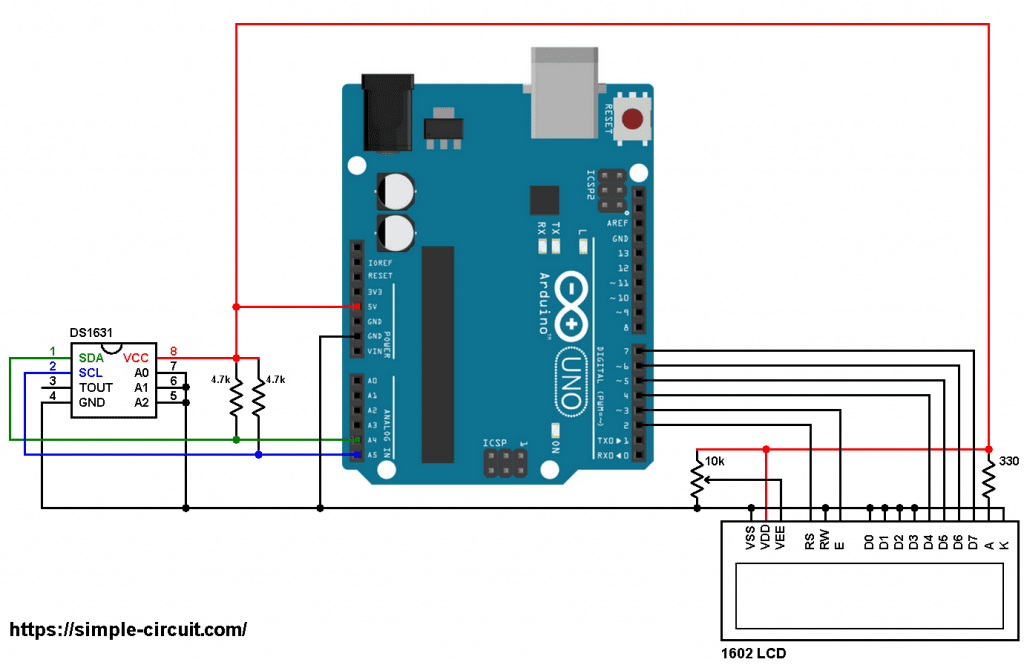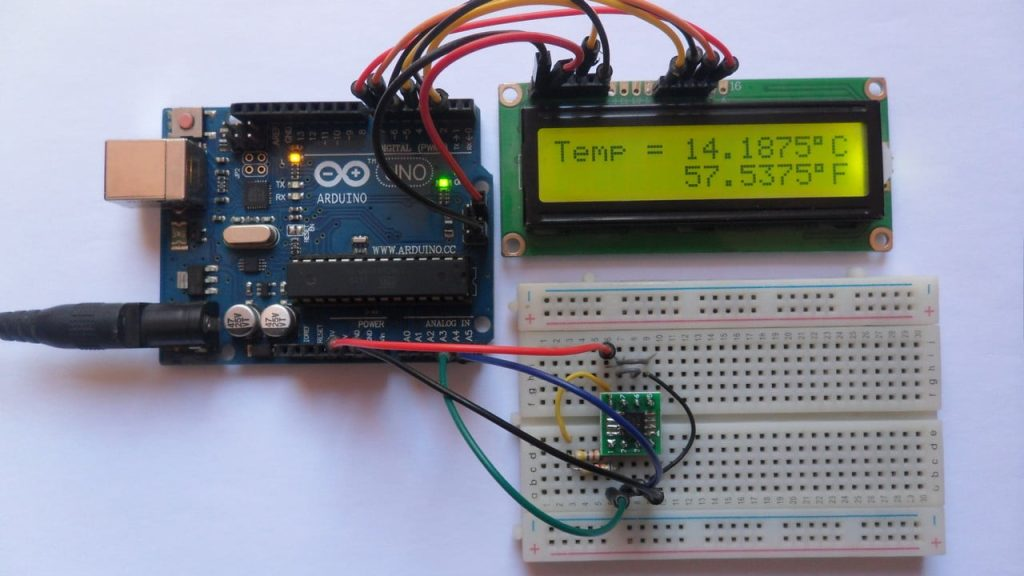# Arduino with DS1631 Temperature Sensor and LCD

This Arduino project shows how to build a simple temperature measurement station with DS1631 digital temperature sensor where temperature values (in degrees Celsius and degrees Fahrenheit) are printed on 1602 LCD screen.

The DS1631 digital thermometer and thermostat from Maxim Integrated provide 9, 10, 11, or 12-bit temperature readings over a -55 °C to +12 °C range. The DS1631 thermometer accuracy is ±0.5 °C from 0 °C to +70 °C with 3.0V ≤ VDD ≤ 5.5V. The thermostat on DS1631 provides custom hysteresis with user-defined trip points (TH and TL). The TH and TL registers and thermometer configuration settings are stored in NV EEPROM so they can be programmed prior to installation.

Benefits and features:

• Operating temperature range: -55 °C to +125 °C (-67 °F to +257 °F).
• ±0.5 °C Accuracy over 0 °C to +70 °C range.
• User-Selectable output resolution from 9 bits to 12 bits.
• Reduce cost with no external components.
• Up to eight devices can operate on a 2-wire bus.
• Flexible and nonvolatile user-defined thermostatic modes with custom hysteresis.
• Available in 8-Pin μSOP, SO and DIP packages.Hardware Required:

• Arduino board
• 1602 LCD screen
• DS1631 temperature sensor   —->   datasheet
• 2 x 4.7k ohm resistor
• 330 ohm resistor
• 10k ohm variable resistor or potentiometer
• Jumper wires

Arduino with DS1631 sensor and 1602 LCD circuit:
Project circuit diagram is shown below.The 1602 LCD screen (2 rows and 16 columns) is used to display temperature values in degrees Celsius and degrees Fahrenheit where:
RS —> Arduino digital pin 2
E   —> Arduino digital pin 3
D4 —> Arduino digital pin 4
D5 —> Arduino digital pin 5
D6 —> Arduino digital pin 6
D7 —> Arduino digital pin 7
VSS, RW, D0, D1, D2, D3 and K are connected to Arduino GND,
VEE to the 10k ohm variable resistor (or potentiometer) output,
VDD to Arduino 5V and A to Arduino 5V through 330 ohm resistor.

VEE pin is used to control the contrast of the LCD. A (anode) and K (cathode) are the back light LED pins.

The DS1631 temperature sensor SDA (serial data) and SCL (serial clock) pins are respectively connected to Arduino A4 and A5 pins (ATmega328P hardware I2C module pins). The DS1631 is supplied with 5V from the Arduino board.
The three pins: A0, A1 and A2 are slave address select pins which are connected to ground, therefore the DS1631 takes an address of 0x48 (1001+A2+A1+A0).

Arduino with DS1631 sensor and 1602 LCD code:
Hints:
Since the address pins of the DS1631 (A2, A1 and A0) are connected to Arduino GND, its I2C slave address is 0x48 which is defined in the code as shown below:

In the Arduino code below there is a function named ds1631_temperature(), it is used to read temperature values from the DS1631 sensor.
The previous function returns a signed 2-byte number which is actual temperature value (in °C) multiplied by 16 (why 16? —> because we’re working with 12-bit resolution).
For example if the returned value equals to 209 means actual temperature is equal to 209/16 = 13.0625 °C.

To get the value of temperature in degrees Fahrenheit (°F), I used the function below:

This function is just from the common one: °F = °C x 9/5 + 32.
Since the temperature in °C is multiplied by 16 the 32 also needs to be multiplied by 16.
To get the °F temperature with no floating number, I multiplied every thing by 10.
This function gives the actual value of the temperature in °F multiplied by 160 (actual °F = f_temp/160).

Since the DS1631 is used with 12-bit resolution, we get the temperature in °C as shown below (example):

Again, why 16 and 625? —> Because we are working with 12-bit resolution (increment step = 0.0625).

The resolution of this thermometer is 0.0625°C (0.1125°F).

Full Arduino code:Arduino with DS1631 sensor and 1602 LCD Proteus simulation:
The following video shows Proteus simulation result of this project.